Next: Worked example 8.6: Horsepower Up: Rotational motion Previous: Worked example 8.4: Weight

## Worked example 8.5: Hinged rod

Question: A uniform rod of mass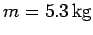and length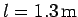rotates about a fixed frictionless pivot located at one of its ends. The rod is released from rest at an angle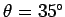beneath the horizontal. What is the angular acceleration of the rod immediately after it is released?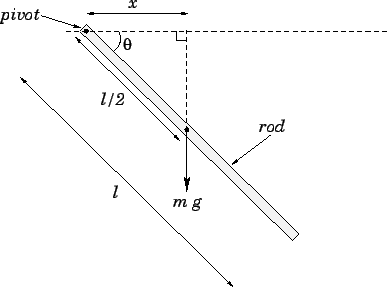Answer: The moment of inertia of a rod of massand length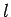about an axis, perpendicular to its length, which passes through one of its ends is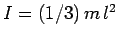(see question 8.3). Hence,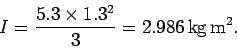The angular equation of motion of the rod is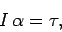where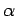is the rod's angular acceleration, and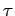is the net torque exerted on the rod. Now, the only force acting on the rod (whose line of action does not pass through the pivot) is the rod's weight,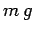. This force acts at the centre of mass of the rod, which is situated at the rod's midpoint. The perpendicular distancebetween the line of action of the weight and the pivot point is simply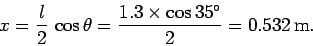Thus, the torque acting on the rod is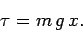It follows that the rod's angular acceleration is written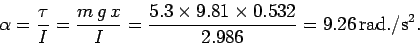Next: Worked example 8.6: Horsepower Up: Rotational motion Previous: Worked example 8.4: Weight
Richard Fitzpatrick 2006-02-02